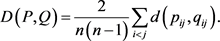# 直觉积性判断矩阵的对数一致性及其检验与修正算法Algorithm to Check and Reach the Log-Consistency of Intuitionistic Multiplicative Judgement Matrices

• 全文下载: PDF(479KB)    PP.107-114   DOI: 10.12677/ORF.2019.91012
• 下载量: 320  浏览量: 908   科研立项经费支持

Intuitionistic multiplicative judgement matrices, which can reflect our intuition more objectively, have been extensively investigated. In this paper, based on the existing work on intuitionistic mul-tiplicative judgement matrices, the consistency of the intuitionistic multiplicative judgement ma-trices is investigated. Concretely, the concept of Log-consistency and its formula of intuitionistic multiplicative matrices is provided; then algorithms to check and modify the Log-consistency of single or several intuitionistic multiplicative judgement matrices are given, which can keep the in-itial judgement information as much as possible.

1. 引言

2. 预备知识

1) 如果 $s\left({\alpha }_{1}\right)>s\left({\alpha }_{2}\right)$ ，那么 ${\alpha }_{1}>{\alpha }_{2}$

2) 如果 $s\left({\alpha }_{1}\right)=s\left({\alpha }_{2}\right)$ ，那么

$d\left({\alpha }_{1},{\alpha }_{2}\right)=\frac{1}{8\mathrm{ln}3}\left(|\mathrm{ln}\frac{{\rho }_{1}}{{\rho }_{2}}|+|\mathrm{ln}\frac{{\sigma }_{1}}{{\sigma }_{2}}|\right).$3. 直觉积性判断矩阵对数一致性及其检验与修正算法

3.1. 直觉积性判断矩阵对数一致性

1) $\mu \left(x,y\right)=\frac{\left(1+x\right)\left(3-y\right)-4}{4-\left(1+x\right)\left(1+y\right)}\in \left[-1,1\right]$

2) $\mu \left(x,y\right)+\mu \left(y,x\right)\le 0$

$\left(1+x\right)\left(3-y\right)-4+4-\left(1+x\right)\left(1+y\right)=2\left(1+x\right)\left(1-y\right)$

$4-\left(1+x\right)\left(1+y\right)-\left(1+x\right)\left(3-y\right)+4=4\left(1-x\right)\ge 0,$

$4-\left(1+x\right)\left(1+y\right)\ge 0$

2) $\mu \left(x,y\right)+\mu \left(y,x\right)=\frac{\left(1+x\right)\left(3-y\right)-4}{4-\left(1+x\right)\left(1+y\right)}+\frac{\left(1+y\right)\left(3-x\right)-4}{4-\left(1+x\right)\left(1+y\right)}=\frac{-2\left(1-x\right)\left(1-y\right)}{4-\left(1+x\right)\left(1+y\right)}\le 0$

$i\left({\mathrm{log}}_{9}{\rho }_{ij}+1\right)\left({\mathrm{log}}_{9}{\rho }_{jk}+1\right)\left({\mathrm{log}}_{9}{\rho }_{ki}+1\right)=\left({\mathrm{log}}_{9}{\rho }_{ji}+1\right)\left({\mathrm{log}}_{9}{\rho }_{kj}+1\right)\left({\mathrm{log}}_{9}{\rho }_{ik}+1\right)$

${\stackrel{^}{\rho }}_{ij}={9}^{\frac{\left(1+{\mathrm{log}}_{9}{\rho }_{i}\right)\left(3-{\mathrm{log}}_{9}{\rho }_{j}\right)-4}{4-\left(1+{\mathrm{log}}_{9}{\rho }_{i}\right)\left(1+{\mathrm{log}}_{9}{\rho }_{j}\right)}}$${\stackrel{^}{\sigma }}_{ij}={9}^{\frac{\left(1+{\mathrm{log}}_{9}{\rho }_{j}\right)\left(3-{\mathrm{log}}_{9}{\rho }_{i}\right)-4}{4-\left(1+{\mathrm{log}}_{9}{\rho }_{i}\right)\left(1+{\mathrm{log}}_{9}{\rho }_{j}\right)}}$$i\ne j$${\stackrel{^}{\rho }}_{ii}={\stackrel{^}{\sigma }}_{ii}=1$ ，为直觉积性对数一致性判断矩阵。

$\begin{array}{l}\left({\mathrm{log}}_{9}{\stackrel{^}{\rho }}_{ij}+1\right)\left({\mathrm{log}}_{9}{\stackrel{^}{\rho }}_{jk}+1\right)\left({\mathrm{log}}_{9}{\stackrel{^}{\rho }}_{ki}+1\right)\\ =\frac{\left(1+{\mathrm{log}}_{9}{\rho }_{i}\right)\left(2-2{\mathrm{log}}_{9}{\rho }_{j}\right)}{4-\left(1+{\mathrm{log}}_{9}{\rho }_{i}\right)\left(1+{\mathrm{log}}_{9}{\rho }_{j}\right)}\frac{\left(1+{\mathrm{log}}_{9}{\rho }_{j}\right)\left(2-2{\mathrm{log}}_{9}{\rho }_{k}\right)}{4-\left(1+{\mathrm{log}}_{9}{\rho }_{j}\right)\left(1+{\mathrm{log}}_{9}{\rho }_{k}\right)}\frac{\left(1+{\mathrm{log}}_{9}{\rho }_{k}\right)\left(2-2{\mathrm{log}}_{9}{\rho }_{i}\right)}{4-\left(1+{\mathrm{log}}_{9}{\rho }_{k}\right)\left(1+{\mathrm{log}}_{9}{\rho }_{i}\right)}\\ =\frac{\left(1+{\mathrm{log}}_{9}{\rho }_{j}\right)\left(2-2{\mathrm{log}}_{9}{\rho }_{i}\right)}{4-\left(1+{\mathrm{log}}_{9}{\rho }_{i}\right)\left(1+{\mathrm{log}}_{9}{\rho }_{j}\right)}\frac{\left(1+{\mathrm{log}}_{9}{\rho }_{k}\right)\left(2-2{\mathrm{log}}_{9}{\rho }_{j}\right)}{4-\left(1+{\mathrm{log}}_{9}{\rho }_{j}\right)\left(1+{\mathrm{log}}_{9}{\rho }_{k}\right)}\frac{\left(1+{\mathrm{log}}_{9}{\rho }_{i}\right)\left(2-2{\mathrm{log}}_{9}{\rho }_{k}\right)}{4-\left(1+{\mathrm{log}}_{9}{\rho }_{k}\right)\left(1+{\mathrm{log}}_{9}{\rho }_{i}\right)}\\ =\left({\mathrm{log}}_{9}{\stackrel{^}{\rho }}_{ji}+1\right)\left({\mathrm{log}}_{9}{\stackrel{^}{\rho }}_{kj}+1\right)\left({\mathrm{log}}_{9}{\stackrel{^}{\rho }}_{ik}+1\right)\end{array}$

3.2. 单个直觉积性判断矩阵对数一致性判断与修正算法

$P=\left(\begin{array}{llll}\left(1,1\right)\hfill & \left(2,2/5\right)\hfill & \left(1/4,5/3\right)\hfill & \left(1/2,2\right)\hfill \\ \left(2/5,2\right)\hfill & \left(1,1\right)\hfill & \left(2,1/3\right)\hfill & \left(8/7,1/3\right)\hfill \\ \left(5/3,1/4\right)\hfill & \left(1/3,2\right)\hfill & \left(1,1\right)\hfill & \left(5,1/7\right)\hfill \\ \left(2,1/2\right)\hfill & \left(1/3,8/7\right)\hfill & \left(1/7,5\right)\hfill & \left(1,1\right)\hfill \end{array}\right),$

$\omega =\left(\left(1.06,0.915\right),\left(2.218,0.415\right),\left(3.238,0.309\right),\left(1.029,0.966\right)\right)$

$\stackrel{^}{P}=\left(\begin{array}{llll}\left(1,1\right)\hfill & \left(0.\text{336},\text{1}.0\text{45}\right)\hfill & \left(0.\text{264},\text{1}.\text{667}\right)\hfill & \left(0.\text{5},\text{}0.\text{48}\right)\hfill \\ \left(\text{1}.0\text{45},\text{}0.\text{336}\right)\hfill & \left(1,1\right)\hfill & \left(0.\text{478},\text{1}.0\text{57}\right)\hfill & \left(\text{1}.0\text{61},\text{}0.\text{328}\right)\hfill \\ \left(\text{1}.\text{667},\text{}0.\text{264}\right)\hfill & \left(\text{1}.0\text{57},\text{}0.\text{478}\right)\hfill & \left(1,1\right)\hfill & \left(\text{1}.\text{691},\text{}0.\text{259}\right)\hfill \\ \left(0.\text{48},\text{}0.\text{5}\right)\hfill & \left(0.\text{328},\text{1}.0\text{61}\right)\hfill & \left(0.\text{259},\text{1}.\text{691}\right)\hfill & \left(1,1\right)\hfill \end{array}\right)$

${d}_{12}=0.312>D,\text{}{d}_{13}=0.006,\text{}{d}_{14}=0.162,\text{}{d}_{23}=0.294>D,\text{}{d}_{24}=0.01,\text{}{d}_{34}=0.191>D$

$\omega =\left(\left(0.98,\text{}0.77\right),\left(2.21,\text{}0.36\right),\left(2.4,\text{}0.29\right),\left(0.95,\text{}0.68\right)\right)$

$\stackrel{^}{P}=\left(\begin{array}{llll}\left(1,1\right)\hfill & \left(0.\text{318},\text{1}.0\text{81}\right)\hfill & \left(0.\text{3}0\text{3},\text{1}.\text{188}\right)\hfill & \left(0.\text{484},\text{}0.\text{462}\right)\hfill \\ \left(\text{1}.0\text{81},\text{}0.\text{318}\right)\hfill & \left(1,1\right)\hfill & \left(0.\text{619},\text{}0.\text{722}\right)\hfill & \left(\text{1}.\text{1},\text{}0.\text{31}\right)\hfill \\ \left(\text{1}.\text{188},\text{}0.\text{3}0\text{3}\right)\hfill & \left(0.\text{722},\text{}0.\text{619}\right)\hfill & \left(1,1\right)\hfill & \left(\text{1}.\text{2}0\text{9},\text{}0.\text{296}\right)\hfill \\ \left(0.\text{462},\text{}0.\text{484}\right)\hfill & \left(0.\text{31},\text{1}.\text{1}\right)\hfill & \left(0.\text{296},\text{1}.\text{2}0\text{9}\right)\hfill & \left(1,1\right)\hfill \end{array}\right)$

$\stackrel{˜}{P}=\left(\begin{array}{llll}\left(1,1\right)\hfill & \left(0.\text{318},\text{1}.0\text{68}\right)\hfill & \left(1/4,5/3\right)\hfill & \left(1/2,2\right)\hfill \\ \left(\text{1}.0\text{68},0.\text{318}\right)\hfill & \left(1,1\right)\hfill & \left(0.\text{62}0,\text{}0.\text{722}\right)\hfill & \left(8/7,1/3\right)\hfill \\ \left(5/3,1/4\right)\hfill & \left(0.\text{722},0.\text{62}0\right)\hfill & \left(1,1\right)\hfill & \left(\text{1}.\text{2}09,\text{}0.\text{2957}\right)\hfill \\ \left(2,1/2\right)\hfill & \left(1/3,8/7\right)\hfill & \left(0.\text{2957},\text{1}.\text{2}09\right)\text{}\hfill & \left(1,1\right)\hfill \end{array}\right),$

3.3. 多个直觉积性判断矩阵对数一致性检验与修正算法

${P}_{1}=\left(\begin{array}{llll}\left(1,1\right)\hfill & \left(2,2/5\right)\hfill & \left(1/4,5/3\right)\hfill & \left(1/2,2\right)\hfill \\ \left(2/5,2\right)\hfill & \left(1,1\right)\hfill & \left(2,1/3\right)\hfill & \left(8/7,1/3\right)\hfill \\ \left(5/3,1/4\right)\hfill & \left(1/3,2\right)\hfill & \left(1,1\right)\hfill & \left(5,1/7\right)\hfill \\ \left(2,1/2\right)\hfill & \left(1/3,8/7\right)\hfill & \left(1/7,5\right)\hfill & \left(1,1\right)\hfill \end{array}\right),$

${P}_{2}=\left(\begin{array}{llll}\left(1,1\right)\hfill & \left(1/2,6/5\right)\hfill & \left(1,2/3\right)\hfill & \left(1/7,3\right)\hfill \\ \left(6/5,1/2\right)\hfill & \left(1,1\right)\hfill & \left(2,1/5\right)\hfill & \left(1/4,2/3\right)\hfill \\ \left(2/3,1\right)\hfill & \left(1/5,2\right)\hfill & \left(1,1\right)\hfill & \left(3/4,1\right)\hfill \\ \left(3,1/7\right)\hfill & \left(2/3,1/4\right)\hfill & \left(1,3/4\right)\hfill & \left(1,1\right)\hfill \end{array}\right),$

${P}_{3}=\left(\begin{array}{llll}\left(1,1\right)\hfill & \left(1/3,3/2\right)\hfill & \left(1,3/5\right)\hfill & \left(1/3,3\right)\hfill \\ \left(3/2,1/3\right)\hfill & \left(1,1\right)\hfill & \left(2,2/5\right)\hfill & \left(1,6/7\right)\hfill \\ \left(3/5,1\right)\hfill & \left(2/5,2\right)\hfill & \left(1,1\right)\hfill & \left(1/3,2\right)\hfill \\ \left(3,1/3\right)\hfill & \left(6/7,1\right)\hfill & \left(2,1/3\right)\hfill & \left(1,1\right)\hfill \end{array}\right)$

$\omega =\left(\left(2.016,0.184\right),\left(4.226,0.163\right),\left(2.016,0.29\right),\left(3.738,0.243\right)\right)$

$\stackrel{^}{P}=\left(\begin{array}{llll}\left(1,1\right)\hfill & \left(0.\text{333},\text{1}.\text{7}0\text{2}\right)\hfill & \left(0.\text{637},\text{}0.\text{637}\right)\hfill & \left(0.\text{379},\text{1}.\text{4}0\text{1}\right)\hfill \\ \left(\text{1}.\text{7}0\text{2},\text{}0.\text{333}\right)\hfill & \left(1,1\right)\hfill & \left(\text{1}.\text{7}0\text{2},\text{}0.\text{333}\right)\hfill & \left(0.\text{959},\text{}0.\text{667}\right)\hfill \\ \left(0.\text{637},\text{}0.\text{637}\right)\hfill & \left(0.\text{333},\text{1}.\text{7}0\text{2}\right)\hfill & \left(1,1\right)\hfill & \left(0.\text{379},\text{1}.\text{4}0\text{1}\right)\hfill \\ \left(\text{1}.\text{4}0\text{1},\text{}0.\text{379}\right)\hfill & \left(0.\text{667},\text{}0.\text{959}\right)\hfill & \left(\text{1}.\text{4}0\text{1},\text{}0.\text{379}\right)\hfill & \left(1,1\right)\hfill \end{array}\right)$

$D\left({P}_{1},\stackrel{^}{P}\right)=0.221$$D\left({P}_{2},\stackrel{^}{P}\right)=0.\text{114}$$D\left({P}_{3},\stackrel{^}{P}\right)=0.0\text{5}$

${d}_{121}=0.369>D,{d}_{131}=0.216>D,{d}_{141}=0.072,{d}_{231}=0.018,{d}_{241}=0.099,{d}_{341}=0.553>D$

$\omega =\left(\left(1.454,0.374\right),\left(3.503,0.143\right),\left(1.305,0.755\right),\left(3.172,0.293\right)\right)$

$\stackrel{^}{P}=\left(\begin{array}{llll}\left(1,1\right)\hfill & \left(0.\text{3}0\text{9},\text{1}.\text{571}\right)\hfill & \left(0.\text{597},\text{}0.\text{5}0\text{8}\right)\hfill & \left(0.\text{334},\text{1}.\text{369}\right)\hfill \\ \left(\text{1}.\text{571},\text{}0.\text{3}0\text{9}\right)\hfill & \left(1,1\right)\hfill & \left(\text{1}.\text{668},\text{}0.\text{286}\right)\hfill & \left(0.\text{856},\text{}0.\text{668}\right)\hfill \\ \left(0.\text{5}0\text{8},\text{}0.\text{597}\right)\hfill & \left(0.\text{286},\text{1}.\text{668}\right)\hfill & \left(1,1\right)\hfill & \left(0.\text{3}0\text{8},\text{1}.\text{456}\right)\hfill \\ \left(\text{1}.\text{369},\text{}0.\text{334}\right)\hfill & \left(0.\text{668},\text{}0.\text{856}\right)\hfill & \left(\text{1}.\text{456},\text{}0.\text{3}0\text{8}\right)\hfill & \left(1,1\right)\hfill \end{array}\right)$

${\stackrel{˜}{P}}_{1}=\left(\begin{array}{llll}\left(1,1\right)\hfill & \left(0.\text{3}09,\text{1}.\text{58}\right)\hfill & \left(0.\text{597},\text{}0.\text{5}0\text{8}\right)\hfill & \left(1/2,2\right)\hfill \\ \left(\text{1}.\text{58},0.\text{3}09\right)\hfill & \left(1,1\right)\hfill & \left(2,1/3\right)\hfill & \left(8/7,1/3\right)\hfill \\ \left(0.\text{5}0\text{8},0.\text{597}\right)\hfill & \left(1/3,2\right)\hfill & \left(1,1\right)\hfill & \left(0.\text{3}0\text{8,1}.\text{5}0\text{6}\right)\hfill \\ \left(2,1/2\right)\hfill & \left(1/3,8/7\right)\hfill & \left(\text{1}.\text{5}0\text{6},0.\text{3}0\text{8}\right)\hfill & \left(1,1\right)\hfill \end{array}\right),$

$D\left({\stackrel{˜}{P}}_{1},\stackrel{^}{P}\right)=0.041$$D\left({P}_{2},\stackrel{^}{P}\right)=0.\text{118}$$D\left({P}_{3},\stackrel{^}{P}\right)=0.0\text{55}$

${d}_{122}=0.086,{d}_{132}=0.09,{d}_{142}=0.186>D,{d}_{232}=0.061,{d}_{242}=0.14>D,\text{}{d}_{342}=0.144>D$

$\omega =\left(\left(1.17,0.642\right),\left(3.103,0.188\right),\left(0.677,1.478\right),\left(1.916,0.512\right)\right)$

$\stackrel{^}{P}=\left(\begin{array}{llll}\left(1,1\right)\hfill & \left(0.\text{29},\text{1}.\text{499}\right)\hfill & \left(0.\text{657},\text{}0.\text{326}\right)\hfill & \left(0.\text{395},\text{}0.\text{842}\right)\hfill \\ \left(\text{1}.\text{499},\text{}0.\text{29}\right)\hfill & \left(1,1\right)\hfill & \left(\text{1}.\text{916},\text{}0.\text{21}\right)\hfill & \left(\text{1}.\text{111},\text{}0.\text{431}\right)\hfill \\ \left(0.\text{326},\text{}0.\text{657}\right)\hfill & \left(0.\text{21},\text{1}.\text{916}\right)\hfill & \left(1,1\right)\hfill & \left(0.\text{264},\text{1}.0\text{93}\right)\hfill \\ \left(0.\text{842},\text{}0.\text{395}\right)\hfill & \left(0.\text{431},\text{1}.\text{111}\right)\hfill & \left(\text{1}.0\text{93},\text{}0.\text{264}\right)\hfill & \left(1,1\right)\hfill \end{array}\right)$

${\stackrel{˜}{P}}_{2}=\left(\begin{array}{llll}\left(1,1\right)\hfill & \left(1/2,6/5\right)\hfill & \left(1,2/3\right)\hfill & \left(0.\text{395},\text{}0.\text{837}\right)\hfill \\ \left(6/5,1/2\right)\hfill & \left(1,1\right)\hfill & \left(2,1/5\right)\hfill & \left(\text{1}.\text{11}1,\text{}0.\text{431}\right)\hfill \\ \left(2/3,1\right)\hfill & \left(1/5,2\right)\hfill & \left(1,1\right)\hfill & \left(0.\text{264},\text{1}.0\text{9}3\right)\hfill \\ \left(0.\text{837},0.\text{395}\right)\hfill & \left(0.\text{431},\text{1}.\text{11}1\right)\hfill & \left(\text{1}.0\text{9}3,0.\text{264}\right)\hfill & \left(1,1\right)\hfill \end{array}\right),$

$D\left({\stackrel{˜}{P}}_{1},\stackrel{^}{P}\right)=0.0\text{57}$$D\left({\stackrel{˜}{P}}_{2},\stackrel{^}{P}\right)=0.0\text{38}$$D\left({P}_{3},\stackrel{^}{P}\right)=0.0\text{93}$

4. 小结

  Saaty, T.L. (1980) Multicriteria Decision Making: The Analytic Hierarchy Process. McGraw-Hill, New York.  Orlovsky, S.A. (1978) Decision-Making with a Fuzzy Preference Relation. Fuzzy Sets and Systems, 1, 155-167. https://doi.org/10.1016/0165-0114(78)90001-5  Tanino, T. (1984) Fuzzy Preference Orderings in Group De-cision Making. Fuzzy Sets and Systems, 12, 117-131. https://doi.org/10.1016/0165-0114(84)90032-0  Szmidt, E. and Kacprzyk, J. (2003) A Consensus-Reaching Process under Intuitionistic Fuzzy Preference Relations. International Journal of Intelligent Systems, 18, 837-852. https://doi.org/10.1002/int.10119  Xu, Z. (2007) Intuitionistic Preference Relations and Their Application in Group Decision Making. Information Sciences, 177, 2363-2379. https://doi.org/10.1016/j.ins.2006.12.019  Xu, Z. (2007) Intuitionistic Fuzzy Aggregation Operators. IEEE Transactions on Fuzzy Systems, 15, 1179-1187. https://doi.org/10.1109/TFUZZ.2006.890678  Liao, H.C. and Xu, Z. (2016) An Enhanced Consensus Reach-ing Process in Group Decision Making with Intuitionistic Fuzzy Preference Relations. Information Sciences, 329, 274-286. https://doi.org/10.1016/j.ins.2015.09.024  Wan, S., Xu, G. and Dong, J. (2016) A Novel Method for Group Decision Making with Interval-Valued Atanassov Intuitionistic Fuzzy Preference Relations. Information Sciences, 372, 53-71. https://doi.org/10.1016/j.ins.2016.08.019  Xu, Z. and Liao, H. (2015) A Survey of Approaches to Decision Making with Intuitionistic Fuzzy Preference Relations. Knowledge-Based Systems, 80, 131-142. https://doi.org/10.1016/j.knosys.2014.12.034  Xia, M., Xu, Z. and Liao, H. (2013) Preference Relations Based on Intuitionistic Multiplicative Information. IEEE Transactions on Fuzzy Systems, 21, 113-133. https://doi.org/10.1109/TFUZZ.2012.2202907  Jiang, Y., Xu, Z. and Yu, X. (2013) Compatibility Measures and Consensus Models for Group Decision Making with Intuitionistic Multiplicative Preference Relations. Applied Soft Computing, 13, 2075-2086. https://doi.org/10.1016/j.asoc.2012.11.007  Qian, W. and Niu, L. (2016) Intuitionistic Multiplicative Preference Relation and Its Application in Group Decision Making. Journal of Intelligent & Fuzzy Systems, 30, 2859-2870. https://doi.org/10.3233/IFS-151836  Xia, M. and Xu, Z. (2013) Group Decision Making Based on Intuition-istic Multiplicative Aggregation Operators. Applied Mathematical Modelling, 37, 5120-5133. https://doi.org/10.1016/j.apm.2012.10.029  Yu, D. and Fang, L. (2014) Intuitionistic Multiplicative Aggrega-tion Operators with Their Application in Group Decision Making. Journal of Intelligent & Fuzzy Systems, 27, 131-142.  Ren, P., Xu, Z. and Liao, H. (2016) Intuitionistic Multiplicative Analytic Hierarchy Process in Group Decision Making. Computers & Industrial Engineering, 101, 513-524. https://doi.org/10.1016/j.cie.2016.09.025  Zhang, Z. and Pedrycz, W. (2018) Intuitionistic Multiplicative Group Analytic Hierarchy Process and Its Use in Multicriteria Group Decision-Making. IEEE Transactions on Cybernetics, 48, 1950-1962. https://doi.org/10.1109/TCYB.2017.2720167  Xu, Z. (2013) Priority Weight Intervals Derived from Intui-tionistic Multiplicative Preference Relations. IEEE Transactions on Fuzzy Systems, 21, 642-654. https://doi.org/10.1109/TFUZZ.2012.2226893